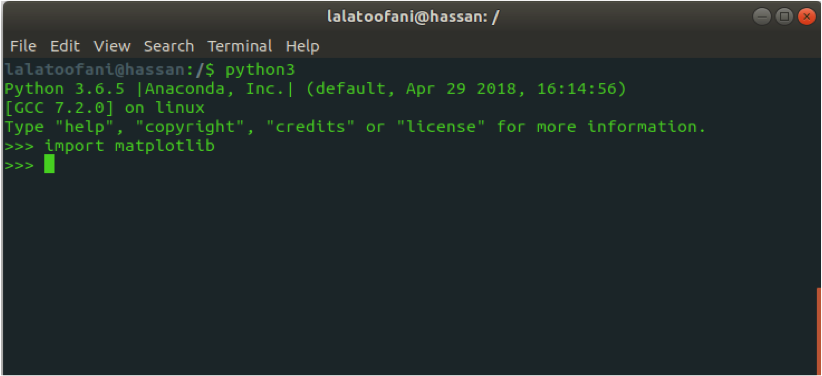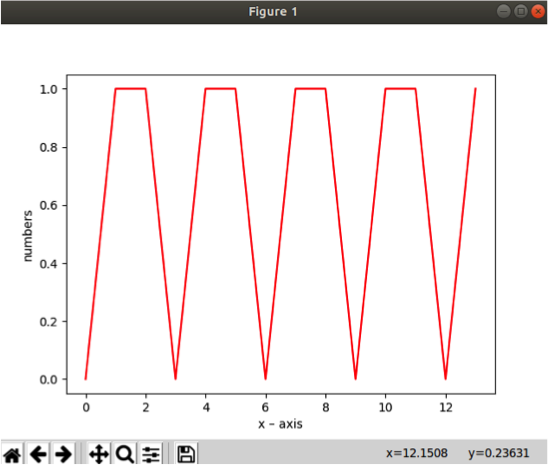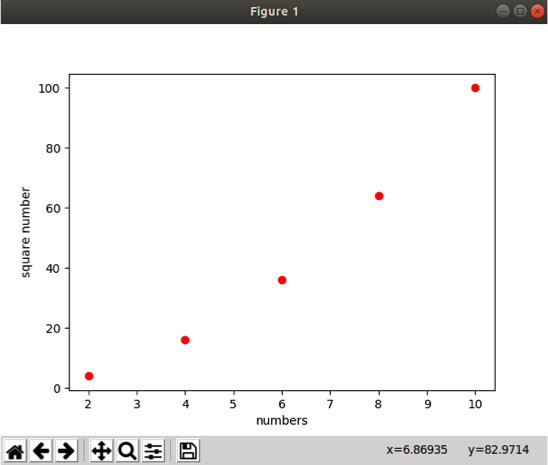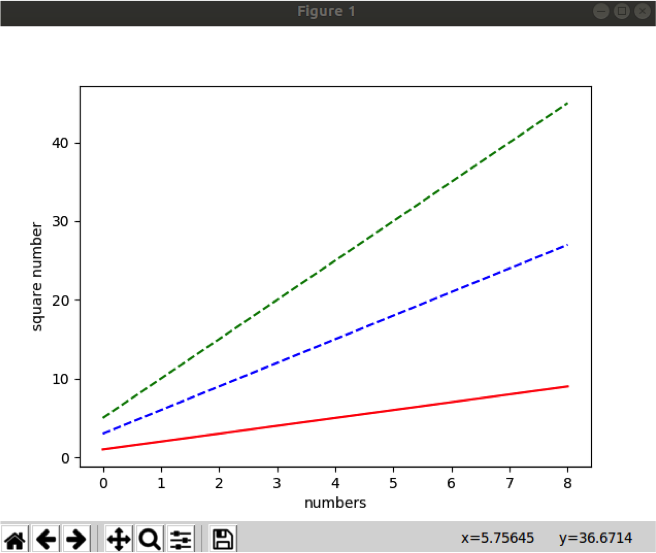## Python Tutorials: Plotting Graph with Matplotlib in Python

##### Python Tutorials: In this part of Learning Python we Cover Plotting Graph with Matplotlib Python
Written by |20-Oct-2020 | 0 Comments | 4189 Views

It is easy to understand the data when it is visualized beautifully. Microsoft has its separate software known as Microsoft excel, which is extensively used for its mathematical calculation and visualizing the data by just one click.

There are lots and lots of websites like Stock Exchange websites or cryptocurrency websites that show the market trend with the help of a graph because the data is so vast that it is not possible to understand by writing in a column.

In python, Matplotlib is the module that is used to visualize the data beautifully. It is the basic module of all new visualizing toolkit.

Not just can you plot a graph of data ranging from one point to the other, but also you can plot the pixel of an image, and even on a higher level, we will see we can plot the medical images which are present in the form of slices.

In this article, we will learn Matplotlib as the beginner level by just using lists and the normal function of python. At a higher level, after learning pandas and NumPy, we will see how to plot more dynamical graphs.

Installation of Matplotlib:

We have to install the Matplotlib by using the pip command as it does not come pre-installed like some of the modules. To install Matplotlib, you have to open your terminal or a command prompt and write the following command:

 pip3 install matplotlib

After running this command, open your python IDE and import Matplotlib. If it imports without giving any error, then it is fine else to try again to install Matplotlib.Figure 1

As mention in figure 1, the import line does not give any error, which indicates it is installed, and we are good to go.

Plotting a simple graph:

To plot a simple graph, we need some information or data set that is to be represented. Initially, we will take the data in the form of the list, but it can be considered as the NumPy array or pandas data frame.

Following is the method to plot a simple graph of 1 and 0 numbers in the list as the data set.

 Code import matplotlib.pyplot as plt plt.plot([0, 1, 1, 0, 1, 1, 0, 1, 1, 0, 1, 1, 0, 1], 'r-') plt.ylabel( 'numbers' ) plt.xlabel( 'x – axis' ) plt.show()

In the above code, we first import the Matplotlib pyplot class. It is convenient to call the module as plt; otherwise, it will be a huge name.

You can name it whatever you want, but I suggest you write it as plt because where ever you see the code, even the original python documentation, they follow the same standard.

We then plot a graph by giving a list of integers as an argument. Here the data is in the range of zero and one. The second argument is r- which indicates that it is the line graph.

In the third and 4th lines, we gave the x and y label their respective name. Moreover, in last we call the show function. If the show function is not called, the code will run fine, but it will not visualize the graph. The following graph will be outputted:Figure 2

As we can see on the y-axis, the number label is printed, and the same in the case of the x-axis, the label is printed. On the y-axis, the range is from 0 to 1 as the data set.

Dot Graph:

There are many types of graphs which we can use according to our needs. Now we will see how to make a dot graph. However, before using it, we must know where we use a dot graph.

Usually, dot graphs are used where we want to mark the point or to make the clusters to see where the density is high and where it is low. Suppose we have to analyze the data of crime of a particular country.

We have data from different cities, and the data is so vast that we cannot understand it by just looking, so we plot the graph according to the location and make the clusters. By these clusters, we can see where the density of crime is high and where it is low.

Following is the code of the dot graph:

 Code import matplotlib.pyplot as plt plt.plot([2,4,6,8,10],[4,16,36,64,100], 'ro') plt.ylabel('square number') plt.xlabel('numbers') plt.show()

In the above code, we have given the three arguments in the plot function. The first list is the points of the x-axis, and the second list is the points of the y-axis.

Here the row indicates the graph is dotted. All the remaining code is the same, as we mentioned above. The following graph is returned as an output:Figure 3

Graphs on the same window:

Sometimes there is a need for applying some functionality on a data and analyze their changes on a graph. In such scenarios, we plot multiple lines on the same graph to know what their changes are concerning the time and increasing functionality.

Now we will plot a graph in which we take data and apply the multiplication of 3 and 5 and see their changes.

 import matplotlib.pyplot as plt data = [1,2,3,4,5,6,7,8,9] plt.plot(data,'r-') plt.plot([i * 3 for i in data],'b--') plt.plot([i * 5 for i in data],'g--') plt.ylabel('square number') plt.xlabel('numbers') plt.show()

In the above example, we plot data with the multiplication of three and five, make the color red, blue, and green. r, b, and g indicate the color. Figure 4 shows the outputted result as a graph.Figure 4

In the above figure 4, the red line indicates the original data, and the blue line indicates the data that is multiplied by the digit three, and the green line shows the data set to multiply by 5.

Saving the Image:

The graph can be saved in the local disk as a png or jpg file. There are two ways to store the image in the local drive.

The one method is to click the floppy disk icon on the window showing the graph. This will save them as an image.

The other way of storing as an image is by calling the same function as follows:

 plt.savefig('imagename.png')